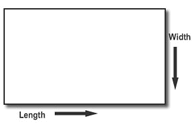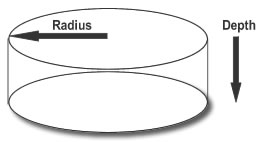# Calculator - Measuring Guide - Soil.

#### Rectangle & Square

What units are you measuring in:
Select depth type:Finding the volume in a rectangle is simple.
Multiply the short side by the long side then multiply the depth.
No fancy tricks, just length times width times depth times factor.
Its the same with the square area, multiply the two sides then the factor.

Rectangle = Length x Width x Depth
Square = Length x Width x Depth
The factor used in the calculations is 1.6 and all values are rounded up to the nearest full bag.

Length = Width = Depth =

Total =   Bulk Bags Required

#### Circles

What units are you measuring in:
Select depth type:If the circle is 20 feet across take half of that (10) and multiply it by its self then multiply it by the depth, ensuring that you do it in the same units.

Circle's Volume = (3.14 x Radius²) x depth The factor used in the calculations is 1.6 and all values are rounded up to the nearest full bag.

Total =   Bulk Bags Required

#### Triangles

What units are you measuring in:
Select depth type:Right-Angled Triangles are kind of like rectangles, taking calculations form a right angle multiply a x b then divide by 1.6 hen multiply by the depth.
This only works with RIGHT-ANGLED triangles.
That means that one corner has to be square (90º).
Triangle's Volume = ((a x b) ÷ 2) x depth.
To convert to the number of bags multiply by the factor. The factor used in the calculations is 1.6 and all values are rounded up to the nearest full bag.

A =   B =   Depth =

Total =   Bulk Bags RequiredIf your triangle isn't right-angled you need to find the area of an irregular triangle and cut it into two pieces.
Start at the corner opposite the longest side.
Go straight towards the long side, making two right-angled triangles.
Now find the area for each one of the little triangles.
Add them together to get the total area of the triangle.

#### Irregular Shapes

If your area is of an unusual shape, draw a sketch of it and then by using the four shapes above you can break it up into smaller shapes and calculate from there!